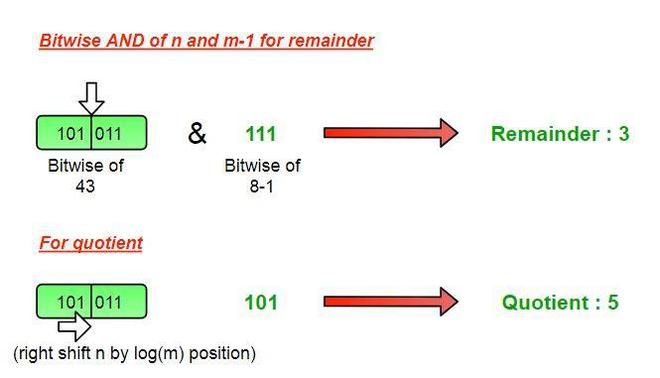GeeksforGeeks App
Open AppBrowser
Continue

# Quotient and remainder dividing by 2^k (a power of 2)

Given a positive integer n as a dividend and another number m (a form of 2^k), find the quotient and remainder without performing actual division

Examples:

Input : n = 43, m = 8
Output : Quotient = 5, Remainder = 3

Input : n = 58, m = 16
Output : Quotient = 3, Remainder = 10

## An approach using bitwise operation

In this, we are using a bitwise representation of a number for understanding the role of division of any number by divisor of form 2^k. All numbers which are power of two include only 1 set bits in their representation and we will use this property.

For finding the remainder we will take logical AND of the dividend (n) and divisor minus 1 (m-1), this will give only the set bits of dividend right to the set bit of divisor which is our actual remainder in that case.

Further, the left part of the dividend (from the position of set bit in divisor) would be considered for quotient. So, from dividend (n) removing all bits right from the position of set bit of divisor will result in the quotient, and right shifting the dividend log2(m) times will do this job for finding the quotient

• Remainder = n & (m-1)
• Quotient = (n >> log2(m) )

Note: Log2(m) will give the number of bits present in the binary representation of m.Finding Quotient and Remainder

Below is the implementation:

## C++

```// CPP to find remainder and quotient
#include<bits/stdc++.h>
using namespace std;

// function to print remainder and quotient
void divide(int n,int m)
{
// print Remainder by
// n AND (m-1)
cout <<"Remainder = " << ((n) &(m-1));

// print quotient by
// right shifting n by (log2(m)) times
cout <<"\nQuotient = " <<(n >> (int)(log2(m)));

}

// driver program
int main()
{
int n = 43, m = 8;
divide(n, m);
return 0;
}

```

## Java

```// Java to find remainder and quotient
import java.io.*;

public class GFG {

// function to print remainder and
// quotient
static void divide(int n, int m)
{

// print Remainder by
// n AND (m-1)
System.out.println("Remainder = "
+ ((n) &(m-1)));

// print quotient by right shifting
// n by (log2(m)) times
System.out.println("Quotient = "
+ (n >> (int)(Math.log(m) / Math.log(2))));
}

// driver program
static public void main (String[] args)
{
int n = 43, m = 8;

divide(n, m);
}
}

// This code is contributed by vt_m.
```

## Python 3

```# Python 3 to find remainder and
# quotient
import math
# function to print remainder and
# quotient
def divide(n, m):

# print Remainder by
# n AND (m-1)
print("Remainder = ",
((n) &(m-1)))

# print quotient by
# right shifting n by
# (log2(m)) times
print("Quotient = " ,(n >>
(int)(math.log2(m))))

# driver program
n = 43
m = 8
divide(n, m)

# This code is contributed by
# Smitha
```

## C#

```
// C# to find remainder and quotient
using System;

public class GFG
{
// function to print remainder and quotient
static void divide(int n,int m)
{
// print Remainder by
// n AND (m-1)
Console.WriteLine("Remainder = " +((n) & (m - 1)));

// print quotient by
// right shifting n by (log2(m)) times
Console.WriteLine("Quotient = "
+ (n >> (int)(Math.Log(m))));

}

// Driver program
static public void Main ()
{
int n = 43, m = 8;
divide(n, m);

}
}

// This code is contributed by vt_m.

```

## PHP

```
<?php
// PHP Code to find remainder
// and quotient

// function to print remainder
// and quotient
function divide(\$n,\$m)
{

// print Remainder by
// n AND (m-1)
echo "Remainder = ". ((\$n) &(\$m - 1));

// print quotient by
// right shifting n by
// (log(m,2)) times 2
// is base
echo "\nQuotient = ".(\$n >> (int)(log(\$m, 2)));

}

// Driver Code
\$n = 43;
\$m = 8;
divide(\$n, \$m);

//This code is contributed by mits
?>

```

## Javascript

```<script>
// javascript program to find last index
// of character x in given string.

// function to print remainder and
// quotient
function divide(n, m)
{

// print Remainder by
// n AND (m-1)
document.write("Remainder = "
+ ((n) &(m-1)) + "<br/>");

// print quotient by right shifting
// n by (log2(m)) times
document.write("Quotient = "
+ (n >> (Math.log(m) / Math.log(2))));
}

// Driver code
let n = 43, m = 8;
divide(n, m);

// This code is contributed by sanjoy_62.
</script>
```
Output

```Remainder = 3
Quotient = 5```

Time Complexity: O(1)
Auxiliary Space: O(1)

My Personal Notes arrow_drop_up